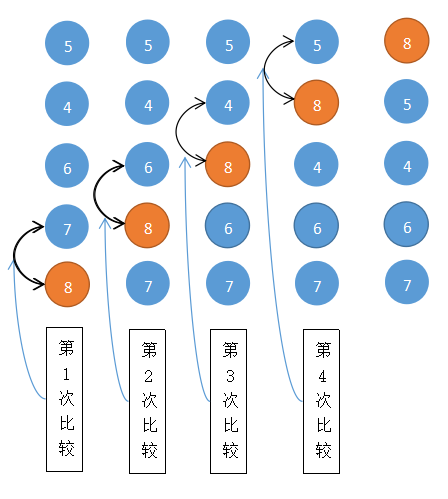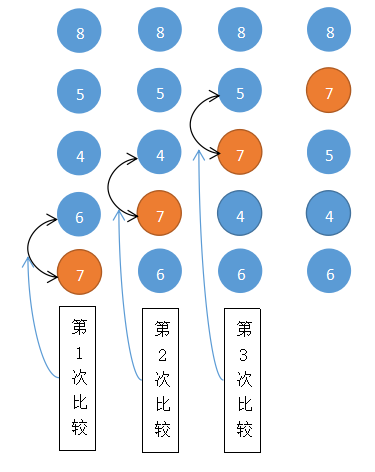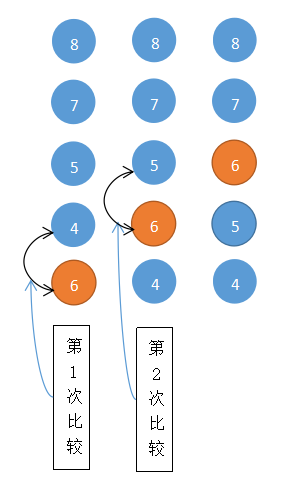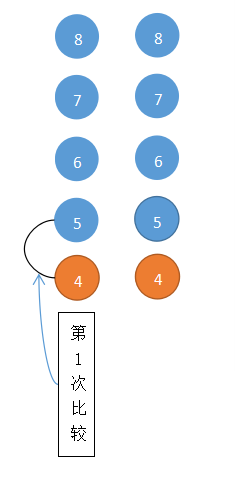# 冒泡排序——《图解算法》

### 一、 图解分析### 二、代码实现

        int[] arr = {8, 7, 6, 4, 5};

for (int i = 0; i < arr.length-1; i++) {

for (int j = 0; j < arr.length - 1 - i; j++) {

if (arr[j] > arr[j + 1]) {
int temp = arr[j];
arr[j] = arr[j + 1];
arr[j + 1] = temp;
}

}

}


### 三、冒泡排序算法的优化

1. 减少外层循环次数的优化

		int[] arr = {8, 7, 6, 1, 2, 3, 4, 5};

// flag: 在一次循环中是否发生过数据交换。true：表示发生过交换，false：表示未发生过交换
boolean flag = true;

for (int i = 0; i < arr.length - 1; i++) {
// 如果未发生交换，则break
if (!flag) {
break;
}
flag = false; // 每次循环都先置为false，即认为不会发生交换
for (int j = 0; j < arr.length - i - 1; j++) {
if (arr[j] > arr[j + 1]) {
flag = true;// 发生了交换
int temp = arr[j];
arr[j] = arr[j + 1];
arr[j + 1] = temp;
}
}
System.out.println(i);
}


2. 减少内层循环次数的优化


int[] arr = {4, 2, 3, 1, 5, 6, 7, 8, 9};

// flag: 在一次循环中是否发生过数据交换。true：表示发生过交换，false：表示未发生过交换
boolean flag = true;

// 上一次内层循环上界值，在第一次循环时与arr.length-1-i相等，即：arr.length-1。也就是说默认是最后一个
int k = arr.length - 1;

for (int i = 0; i < arr.length - 1; i++) {
// 如果未发生交换，则break
if (!flag) {
break;
}

// 每次循环都先置为false，即认为不会发生交换
flag = false;

// 记录上一次内层循环时最后一次交换的位置
int last = 0;

for (int j = 0; j < k; j++) {
if (arr[j] > arr[j + 1]) {
// 发生了交换
flag = true;
// 记录每次交换的位置
last = j;
int temp = arr[j];
arr[j] = arr[j + 1];
arr[j + 1] = temp;
}
}
// 让下一次循环的上界值变成此次循环的最后一次交换的位置
k = last;
}


k为动态值有个好处，即随时动态调整上一次最后交换的位置。这样做的好处是，当遇到数组中有紧挨着的数是连续的时候就会在下一次循环时省略它们的比较。